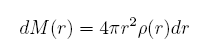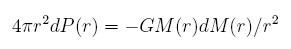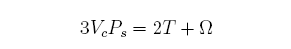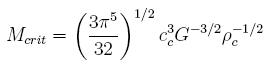Star Formation Rate and the Magnetorotational Instability

Introduction and Historical Background

The distribution of stars is of particular interest to astronomers and has been for some time. Stars are one of our primary probes of the cosmos and understanding their distribution and global structure is therefore of primary importance.  As the scientific community's understanding matured, a coherent picture of stellar lifetime became necessary. With respect to the mechanism by which stars produce fuel, the question was settled in the early 20th century , and it is currently accepted that stars illuminate the universe and prevent their own infall by the process of thermonuclear fusion.

The question of stellar formation, which this note primarily addresses, is one without such simple answers. As early as 1811, Herschel had recognized a correlation between nebulae and young star clusters and hypothesized that the latter emerged from the former.  In the early 20th century, new technologies, such as photographic plates, extended the eyes of the worlds' greatest telescopes. The newfound precision of those measurements led to a necessity of causation from that correlation. Cortie concluded that "unintelligent instruments of Nature can be uniformly directed to a particular end only by an intelligent cause."  Today, these observations are understood as causal, not by some supreme being, but by gravitational collapse of nebulae, or clouds of gas, into stars. The rest of this paper explains that conclusion.

Gravitational Collapse

The main force governing the collapse of a cloud of gas into a star is the cloud's own self-gravity. We consider a massrelating the differential mass of the cloud to its density &rho at some radius r. We are interested in the situation where this cloud of gas collapses (i.e. into a star), and to understand the parameters for collapse, we look at equilibrium behavior. In equilibrium before collapse, Schrödinger pressure holds up the structure with a strength given byIf we integrate this equation in the radial direction, we find that the left hand side is a sum of the volume of the cloud multiplied by the external pressure applied and the thermal energy of the particles in the cloud, multiplied by appropriate constants. The right hand side is the gravitational-self energy of the cloud. Thus, we have an equilibrium relationship between the volume of a cloud and its thermal energy. Mathematically,where Vc is the volume of the cloud, Ps is the external pressure, T is the thermal energy, and &Omega is the gravitational self-energy. 

By calculating the degree of departure from this equilibrium condition, we can estimate the free fall time of such a cloud of gas. We assume initial conditions of a uniformly dense cloud sitting still, with insufficient pressure to counteract self-gravity. The naive calculation reveals a free-fall time ofwhere &rhoc is the density of the cloud. If this free-fall time is less than the cloud-crossing time for sound (i.e. if pressure waves cannot dissipate the energy of collapse), then the cloud of gas will collapse into a proto-star. The free-fall time is a function of density, and the sound-crossing time of the size of the cloud. Therefore, we can isolate the cloud mass. This mass is the critical mass, determining whether or not the cloud will collapse. A little algebra revealswhere cc is the internal sound speed. Any cloud of gas above this critical mass should collapse into a star. We feel accomplished until we notice that Mcrit is around 30 Msun even in the densest molecular clouds, substantially larger than most stars. Some other effects must be at work to create the low-mass stars we see.

The Problem of Angular Momentum

The observed initial mass function of stars can be generated from the above naive calculations by the addition of turbulent fragmentation terms.  When the molecular cloud is struck by a supernova-driven shock wave, the turbulence cascades down to the scale of gravitational collapse and can stimulate such collapse into proto-stellar cores at a different rate than the previous calculation.  These and other such effects perturb our naive approach to something approximating the true initial mass function of stars.  Unfortunately, the ensuing mathematics is not analytically solvable. We construct large scale simulations of a supernova-driven, turbulent interstellar medium with cold, dense molecular clouds, and numerically determine the creation rates of stars from molecular clouds.  Such numerical work may accurately predict the initial mass function of stars, but fails to account for the physics of angular momentum.

Angular momentum conservation suggests that given any angular momentum in the initial gas cloud, as collapse occurs, the average velocity of particles increases. To first order, this acts as a restoring force on the gravitational collapse, and clouds with mass greater than the Jeans mass can survive in equilibrium. If this restoring force was somehow overcome, there would still be an excess of angular momentum in the proto-star, compared to observations.  It is therefore necessary that the angular momentum be transported outward, allowing a smaller mass to hold much of the angular momentum. Consider our solar system today--a substantial fraction of the angular momentum is in Jupiter, even though it is substantially less massive than the sun.

The Magnetorotational Instability

Balbus and Hawley present a mechanism for angular momentum transport.  As the gas cloud collapses, particle collisions collapse it into an accretion disk, whose axis is the direction of net angular momentum. In the presence of a weak magnetic field, the disk is dynamically unstable, and a local shearing force develops. The disk becomes turbulent, with larger centrifugal forces at higher radii, thus increasing the velocity of the gas further out in the disk, and transporting the angular momentum outward. This magnetorotational instability is independent of the magnetic field strength, as long as it is nonzero. 

Recent numerical and analytic work suggest that the magnetorotational instability is capable of transporting angular momentum outward, and that it is the dominant mechanism in protoplanetary disks. The galaxy is pervaded by a weak magnetic field, and thus the physical effect is possible in most molecular clouds.  Unfortunately, as the model increases in complexity, so does the mathematics. The system is fully described by a series of partial differential equations: the continuity equation, the equations of motion for the particles, and the induction equation. However, as with most magnetohydrodynamic problems, little can be fully solved linearly. Salmeron and Wardle make some progress with linearization techniques.  Others rely on complex numerical models, allowing the computer to time-step through the motion of the gas.  Work is continuing to progress in this field, and hopefully a fuller picture will present itself over the ensuing years.

References

 M. F. Tisserand, Bulletin Astronomique, (L'observatoire de Paris et du Bureau des Longitudes, 1884).

 G. Gamow, "A Star Model with Selective Thermo-Nuclear Source," Astrophys. J. 87, 206 (1938).

 A. L. Cortie, "Photographic Evidence for the Formation of Stars from Nebulae," Traill-Taylor Lecture, Royal Photographic Society. 22 (1919).

 J. E. Dyson and D. A. Williams, The Physics of the Interstellar Medium, 2nd Edition, Chapter 8 (Institute of Physics Publishing, 1997).

 A. Nordlund and P. Padoan, "Star Formation and the Initial Mass Function," Turbulence and Magnetic Fields in Astrophysics (Springer, 2003).

 J. Ballesteros-Paredes, E. Vazquez-Semadeni and J. Scalo, "Clouds as Turbulent Density Fluctuations: Implications for Pressure Confinement and Spectral Line Data Interpretation," Astrophys. J. 515, 286 (1999).

 M-M. Mac Low, "MHD Turbulence in Star-Forming Regions and the Interstellar Medium" Turbulence and Magnetic Fields in Astropysics (Springer, 2003).

 K. Tomisaka, "The Evolution of the Angular Momentum Distribution During Star Formation," Astrophys. J. 528, 41 (2000).

 S. A. Balbus and J. F. Hawley, "A Powerful Local Shear Instability in Weakly Magnetized Disks. I Linear Analysis," Astrophys. J. 376, 214 (1991).

 R. Salmeron and M. Wardle, "Magnetorotational Instability in Protoplanetary Dics," Monthly Notices of the Royal Astronomical Society. 361, 45 (2005).

 B. W. Carroll and Dale A. Ostlie, An Introduction to Modern Astrophysics, (Addison-Wesley, 1996).